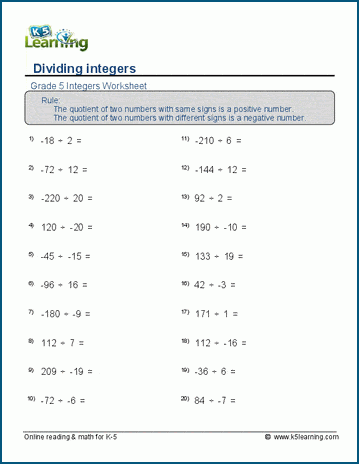# Grade 5 Integer Worksheets

Our grade 5 integer worksheets introduce the addition, subtraction, multiplication and division of negative integers.

## Practice absolute values

The absolute value of an integer is the distance of the integer from zero. The same goes for a negative integer in the opposite direction. Students often practice absolute value on a number line.

In this section, students practice the absolute and opposite value of integers. This is one of the absolute value worksheets: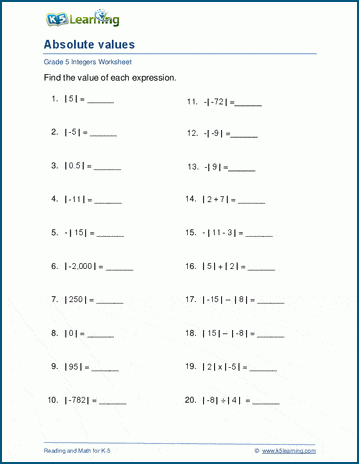## Comparing integers worksheets

These worksheets ask students to compare two integers: whether one integer in greater than, less than or equal to another integer.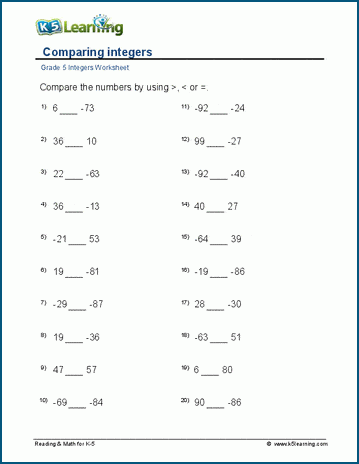A second set of worksheets has students practice putting integers in order.

## Integers on a number line

Practicing integers on a number line is a great way to visualize integers. Students solve integer problems using a number line in these worksheets.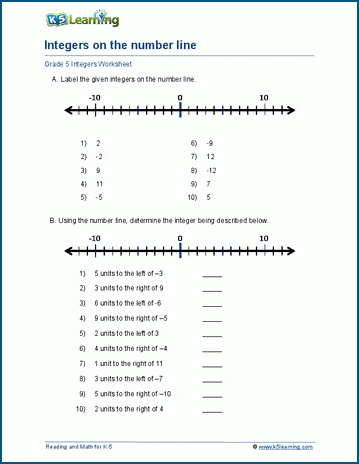## Learn to add negative integers

Students work on adding positive and negative integers in these 2-term, 3-term and vertical addition worksheets. This worksheet has students add a positive and negative integer.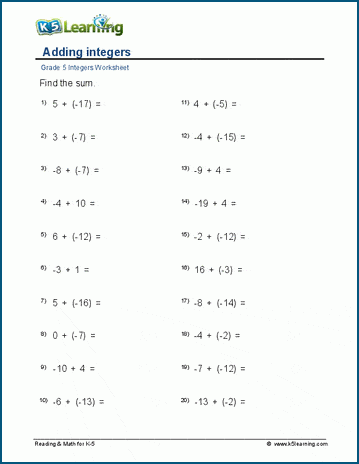## Practice subtracting negative integers

We have two sets of worksheets on subtracting positive and negative integers: numbers under 20 and numbers under 100.

This worksheet tackles subtracting numbers under 20.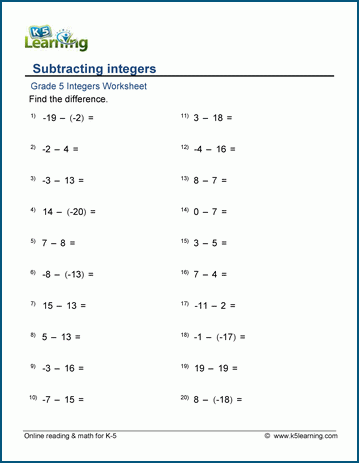## Adding and subtracting integers worksheets

Once students have practiced the addition of integers, then the subtraction of integers, the next step is mixed addition and subtraction of integers.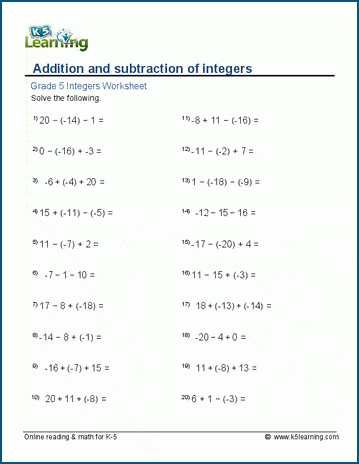## Multiplying negative integers

For students who are ready for the more advanced integer practice, these multiplication of positive and negative integers on the horizontal and vertical are more challenging.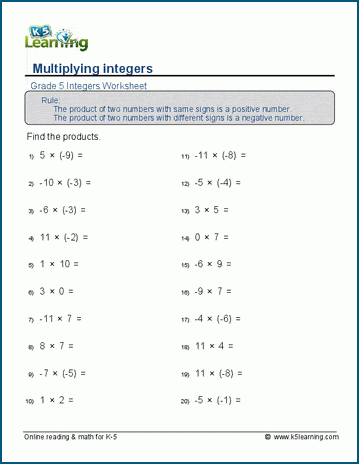## Dividing negative integers practice

The final set of worksheets cover the division of positive and negative integers, horizontally and vertically in long division.